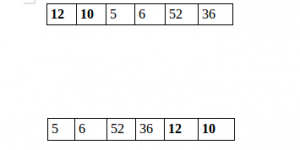Skip to content
Related Articles
C++ Program to Split the array and add the first part to the end
• Difficulty Level : Easy
• Last Updated : 05 Dec, 2018

There is a given an array and split it from a specified position, and move the first part of array add to the end.Examples:

```Input : arr[] = {12, 10, 5, 6, 52, 36}
k = 2
Output : arr[] = {5, 6, 52, 36, 12, 10}
Explanation : Split from index 2 and first
part {12, 10} add to the end .

Input : arr[] = {3, 1, 2}
k = 1
Output : arr[] = {1, 2, 3}
Explanation : Split from index 1 and first
part add to the end.
```

## Recommended: Please try your approach on {IDE} first, before moving on to the solution.

 `// CPP program to split array and move first``// part to end.``#include ``using` `namespace` `std;`` ` `void` `splitArr(``int` `arr[], ``int` `n, ``int` `k)``{``    ``for` `(``int` `i = 0; i < k; i++) {`` ` `        ``// Rotate array by 1.``        ``int` `x = arr;``        ``for` `(``int` `j = 0; j < n - 1; ++j)``            ``arr[j] = arr[j + 1];``        ``arr[n - 1] = x;``    ``}``}`` ` `// Driver code``int` `main()``{``    ``int` `arr[] = { 12, 10, 5, 6, 52, 36 };``    ``int` `n = ``sizeof``(arr) / ``sizeof``(arr);``    ``int` `position = 2;`` ` `    ``splitArr(arr, 6, position);`` ` `    ``for` `(``int` `i = 0; i < n; ++i)``        ``printf``(``"%d "``, arr[i]);`` ` `    ``return` `0;``}`
Output:
```5 6 52 36 12 10
```

Please refer complete article on Split the array and add the first part to the end for more details!

Want to learn from the best curated videos and practice problems, check out the C++ Foundation Course for Basic to Advanced C++ and C++ STL Course for the language and STL. To complete your preparation from learning a language to DS Algo and many more, please refer Complete Interview Preparation Course.
My Personal Notes arrow_drop_up